• 计算傅里叶变换 傅里叶变换 鉴于这种想法，任何信号，当然任何周期性信号，都可以由一系列正弦曲线组成，我们将开始从级数（Series）的概念转向连续信号的概念。我们将要讨论一些东西，这些东西可以让我们知道图像...
目录

傅里叶变换

计算傅里叶变换

傅里叶变换

鉴于这种想法，任何信号，当然任何周期性信号，都可以由一系列正弦曲线组成，我们将开始从级数（Series）的概念转向连续信号的概念。我们将要讨论一些东西，这些东西可以让我们知道图像中任意给定频率的功率有多大。也就是说，我们想把图像从某样东西上变换过来，该东西是时间的函数，或者仅仅是空间的函数，从而知道它的频率是多少。这种变换被称为什么？它叫做傅里叶变换（Fourier Transform）。

现在我们做了傅里叶级数（Fourier series），然后我们进入傅里叶变换（Fourier Transform），再然后我们将进行离散傅里叶变换（Discrete Fourier Transform, DFT），这将使我们得到那个图像的东西。

所以我们想了解频率，频率通常被写成（Omega），我们的信号。我们想要参数化，我们想要变换信号，而不是x，在空间中是通过（Omega）。我们通过所谓的傅里叶变换（Fourier Transform）来实现这一点，傅里叶变换会拿一些空间信号，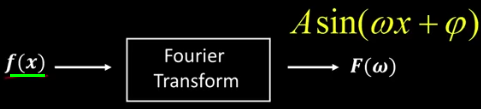并且它将为我们提供一些特性，这些特性可以给每个给定（Omega）的相位 和 幅度进行编码。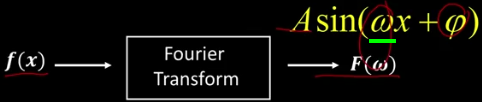好的，所以我们需要给定每个  （Omega）的相位 和 幅度。特别是每个 （Omega）从0到无穷大，实际上它是 从负无穷大到无穷大（到）。这个想法是保存了正弦曲线相应的幅度 和 相位。因此，这个 必须拥有幅度 和 相位。

如何保留振幅和相位？复数的把戏（Complex number trick!）。注意，我没有说过一个单独的数字。因为请记住，只是F的值，所以它是一个数字，将是幅度和相位，我们该怎么做？非常好，实际上，大写字母F实际上是一个复数。现在，希望您能记住关于复数的一些知识。如果没有，我会给你一个非常简短的回顾。所以基本上， 由两部分组成：一个实部（Real Part），另一个是虚部（Imaginary Part）。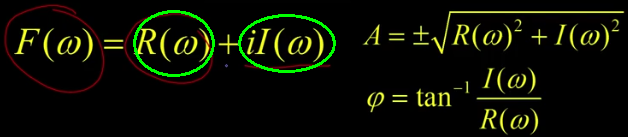所以请记住： ，在这种情况下，  的实部加上虚部，也许你还记得复数的大小就是两个元素之和的平方根。通常是，这里是 。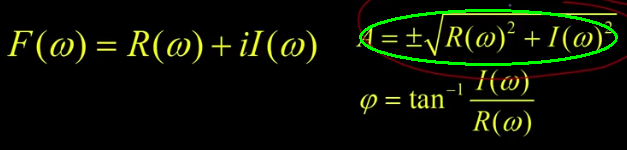代表实部，是虚部，相位之间的关系将以这种方式编写，相位是 虚部 比 实部 的反正切。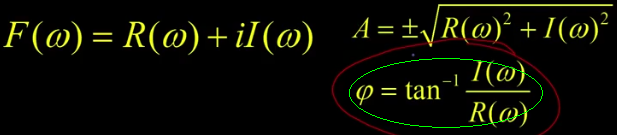这里遗漏的点是：实部归因于 偶函数（Even），虚部归因于 奇函数（Odd），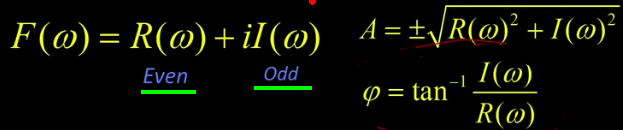所有这意味着：如果我在这里有一个函数，是我的cosign函数，这是关于原点对称的。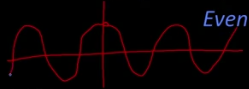正弦曲线，我要把它们拧紧，因为它必须到这里，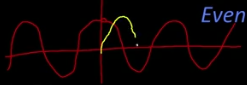然后在那里，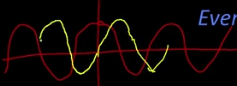再然后，所以它必须是0（zero），在这里，这是我的正弦曲线，因为当那个东西高时，它必须降到0，奇怪的是，Sin (- x) 等于 -Sin (x) ，而 Cos (- x) 等于 Cos (x)。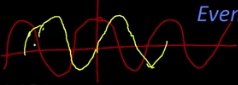所以最后的这个部分（如图），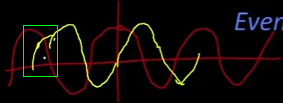实部是余弦部分（Cosin），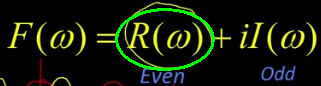虚部是正弦部分（Sine）。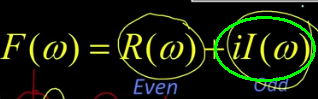计算傅里叶变换

当我们进行傅里叶变换时，我们所做的只是计算基集。我会告诉你我的意思。看到这个丑陋，丑陋的整体吗？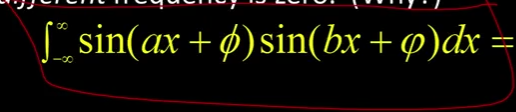好吧，我得到的是，我已经得到了一个函数的正弦值，另一个函数的正弦值。，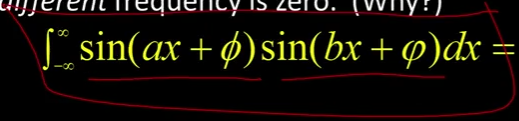两个不同的频率， 和 ，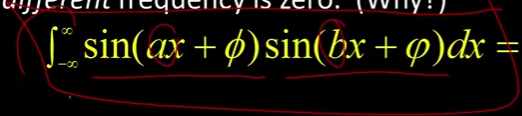我将这一点从负无穷大加到正无穷大，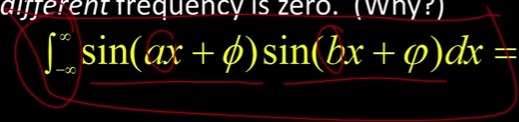我声称如果  不等于 ，它等于0。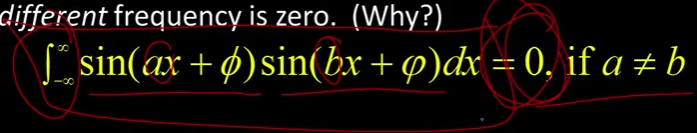为什么这是正确的呢？有猜测吗? 让我们直观地思考一下，如果有一个正弦曲线，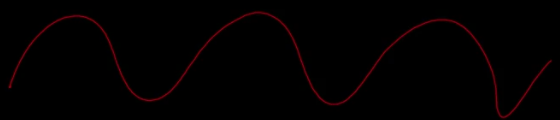现在我必须做一个不同的频率，这很容易，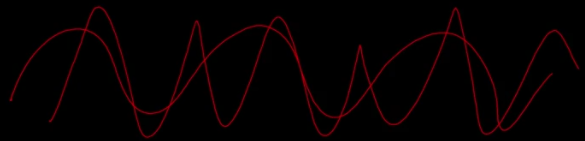天啊，真漂亮！哈哈？ 在本质上，在某个点上，当这个是正的这个是相同的正值，我会试着找到，希望有一些是正的，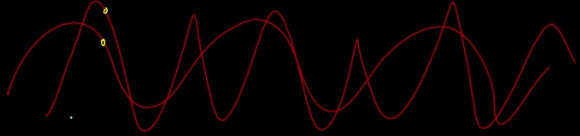我必须补上一个点。对，让我们假装红色的东西在这里下来就像那样 一点点。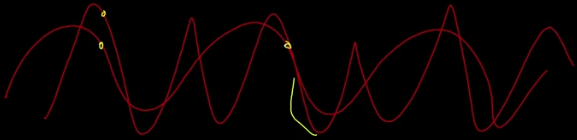当红色的点是正数时，黄色的点是负数，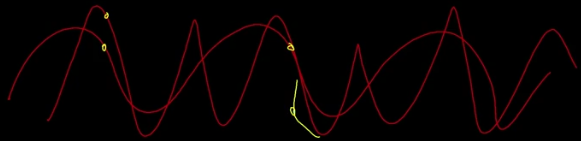所以这两个数的乘积会相互抵消，这是一种波浪的方式表示整个积分是0，只要   。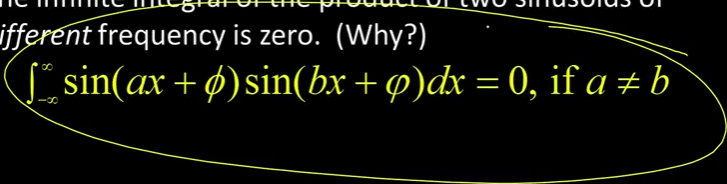但是你可能会问当时会发生什么?

我们先假设它们处于相同的相位，完全相同的相位。好吧，它就是Sin²。Sin²是正的，在无穷远处求和，得到什么？你得到无穷大。所以，如果你有两个频率相同的正弦波，你会得到一个无穷大的值。除非它们完全不相合，在这种情况下是0。换句话说，Sin乘以Cos继续下去。但最基本的思路是，如果我整合一个函数，如果它是由一个与不同的正弦曲线组成的，当我采用的正弦并且我完全间隔时，我什么也得不到。但是如果它是等于那个正弦，我会得到无穷大。而且这将是这样写的。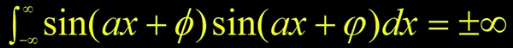所以让我们做一个简单的例子。假设有一个简单的函数：，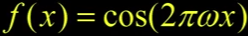然后我们选一些频率，我们把它叫做，简单点。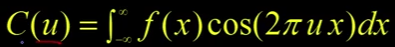如果我取这个积分，Okay。那么如果  等于那个相同的  那将是无穷的，否则它将是零。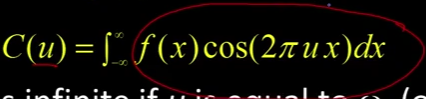所以看起来像这样， Okay。我们只有这两个成员脉冲。这里只有这两个无穷大的尖峰。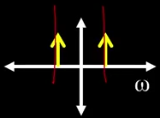这被称为与余弦对应的脉冲。你可以看到它们是正的，这是因为它是余弦。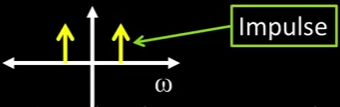如果我们有一个正弦，因为，它看起来就像在这里的向上，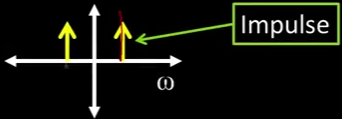和另一个将会在这里向下。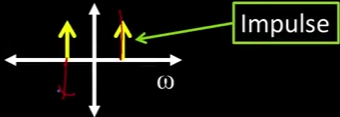这就是正弦，Okay。这就是虚部部分。两个公式如下：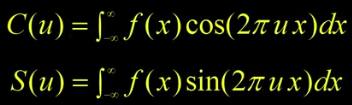我们只是在计算一个基集来说明这个正弦曲线有多少。我们可以为所有频率做到这一点。但是你可能会问，我们不是也必须为所有相位做到这一点吗？

答案是否定的。最酷的事情之一是，如果我有 和 。所以1是90度 或 两相相变的功率。我可以通过它们的线性组合得到任意相位。你可以证明，这是真的，我们可以做一个小演示。但基本上，如果我有一个任意相位的正弦曲线，如果你告诉我它对余弦的积分是多少、它对正弦的积分是多少，我就能告诉你这个正弦曲线的相位是多少。

复数的基础：

https://blog.csdn.net/sw3300255/article/details/83149483

——学会编写自己的代码，才能练出真功夫。

展开全文机器视觉
• FFT是一个用O(nlog_2 n)的时间将一个用系数表示的多项式转换成它的点值表示的算法，其用于加速多项式高精度乘法的时间O(n^2)，是对DFT（离散傅里叶变换）的一个分治的做法。 调用Matlab自带的fft函数进行运算，得出...
傅里叶快速变换 FFT是一个用O(nlog_2 n)的时间将一个用系数表示的多项式转换成它的点值表示的算法，其用于加速多项式高精度乘法的时间O(n^2)，是对DFT（离散傅里叶变换）的一个分治的做法。
调用Matlab自带的fft函数进行运算，得出abs（fft（data-mean（data）））的结果，数据进行了去均值化，减小运算速度，同时data的数量为2的整数次幂，得出频谱图。
最后查出频谱图的最大值的坐标index(应避开零点带来的冲激响应，坐标不能取到零点附近的部分)，代入频率计算公式h=60秒*（index）*采样频率/(length(data))。


展开全文fft
• 用java实现图像的快速傅里叶变换频率域滤波
首先感谢中山大学12级软件学院计算机应用方向和我同班的乔勃大God，以及软件学院副院长、数图seisei朝老师的帮助！马屁还是要拍的o(*￣▽￣*)ブ
//-------------------------------------------------OK 正题---------------------------------------------------------
很多学过数图的小伙伴都经历过傅里叶变换这一坑，如果使用中文书的话错误就更多了。
So，今天小渣我就用java实现一个快速傅里叶变换和频率域的滤波，函数都是纯手写（用来弄懂原理）
废话不多说，先看看频率域滤波的大致步骤：
1、给定一副A*B的图像，对其进行扩充（补0），使其长和宽变为大于或等于自身长度的最小2的整数次幂。
2、对扩充后的图像进行移动中心操作，即乘以（-1）^(x+y)。
3、计算第二步的FFT（利用一维快速傅里叶变换实现二维）【生成一个大小和扩充图像一致的二维复数数组F（u，v）】
4、生成一个频率域的滤波器H（u，v）【注意这个是复数，大小和第三步的结果一样】
5、点乘，令g（u，v）= H（u，v）·F（u，v）
6、对g做快速傅里叶逆变换IFFT
7、对第6步的结果取实部
8、队第7步的结果乘以（-1）^(x+y)再次移动中心。
9、剪裁获得与原图大小一致的结果。

sorry，今天好累，先开个头，把代码放上去，明天继续。
/*   这个文件里包含两个DFT函数，一个是主DFT，在这里完成一个二维图片的
扩充、中心移动、傅里叶变换、滤波（包括滤波器的处理等）、逆变换、取实部、
移回中心等操作并返回一个处理完后的二维图片。另一个DFT是指普通的（慢速）
傅里叶变换，输入一个二维数组、u、v并返回一个值F（u、v）。
除此之外，本文件还提供：
·get2PowerEdge：用来计算一个图像边的最小2的整数次幂扩展；
·showFouriedImage：返回一个傅里叶变换后的频谱图（取模、取log再量化）
·fft：一维快速傅里叶变换
·ifft：一维快速傅里叶逆变换
·convolve：一维卷积。*/

import java.awt.Image;
import java.awt.image.BufferedImage;
import java.io.File;
import java.io.IOException;
import java.lang.reflect.Array;
import java.util.Scanner;
import java.lang.Math;

import javax.imageio.ImageIO;

public class ImageFourier {

public static void main (String[] args) throws IOException {
BufferedImage destimg = DFT(srcimg);
ImageIO.write(destimg, "png", new File("G:/pic/NormalDFT84.png"));
System.out.println("finished!");
}

public static BufferedImage DFT(BufferedImage img) throws IOException {

int w = img.getWidth(null);
int h = img.getHeight(null);
int m = get2PowerEdge(w); // 获得2的整数次幂
//		System.out.println(m);
int n = get2PowerEdge(h);
//		System.out.println(n);
int[][] last = new int[m][n];
Complex[][] next = new Complex[m][n];
int pixel, alpha = -1, newred, newgreen, newblue, newrgb;

BufferedImage destimg = new BufferedImage(w, h, BufferedImage.TYPE_INT_ARGB);
//----------------------------------------------------------------------
//  first: Image Padding and move it to center 填充图像至2的整数次幂并乘以（-1）^(x+y)
//  use 2-D array last to store
for (int i = 0; i < m; i++) {
for (int j = 0; j < n; j++) {
if (i < w && j < h) {
pixel = img.getRGB(i, j);
if ((i+j)%2==0) {
newred = pixel&0x00ff0000>>16;
}
else {
newred = -(pixel&0x00ff0000>>16);
}
last[i][j] = newred;
}
else {
last[i][j] = 0;
}
}
}

//----------------------------------------------------------------------
// second: Fourier Transform 离散傅里叶变换
// u-width v-height x-width y-height

//------------Normal-DFT-------------
//			for (int u = 0; u < m; u++){
//				for (int v = 0; v <n; v++) {
//					next[u][v] = DFT(last, u, v);
//					System.out.println("U: "+u+"---v: "+v);
//				}
//			}
//			if (true) { // 生成DFT图片,记得修改图片大小
//			destimg = showFourierImage(next);
//			return destimg;
//		}

//---------------FFT-----------------
// 先把所有的行都做一维傅里叶变换，再放回去
Complex[] temp1 = new Complex[n];
for (int x = 0; x < m; x++) {
for (int y = 0; y < n; y++) {
Complex c = new Complex(last[x][y],0);
temp1[y] = c;
}
next[x] = fft(temp1);
}

// 再把所有的列（已经被行的一维傅里叶变换所替代）都做一维傅里叶变换
Complex[] temp2 = new Complex[m];
for (int y = 0; y < n; y++) {
for (int x = 0; x < m; x++) {
Complex c = next[x][y];
temp2[x] = c;
}
temp2 = fft(temp2);
for (int i = 0; i < m; i++) {
next[i][y] = temp2[i];
}
}

//		if (true) { // 生成DFT图片,记得修改图片大小
//			destimg = showFourierImage(next);
//			return destimg;
//		}

//----------------------------------------------------------------------
// third: Generate the frequency filter and filter the image in frequency domain 生成频率域滤波器并滤波

// 构造原始滤波函数
Complex[][] filter = new Complex[m][n];
//这个是11X11均值滤波
//		for (int x = 0; x < m; x++) {
//			for (int y = 0; y < n; y++) {
//				if (x < 11 && y < 11) {
//					if ((x+y)%2==0)
//						filter[x][y] = new Complex(1/121d, 0); // double 后面赋值数字记得加d！！！！！！！
//					else
//						filter[x][y] = new Complex(-1/121d, 0);
//				}
//				else {
//					filter[x][y] = new Complex(0, 0);
//				}
//			}
//		}

//下面这个是拉普拉斯滤波
filter = new Complex(0, 0);
filter = new Complex(-1, 0);
filter = new Complex(0, 0);
filter = new Complex(-1, 0);
filter = new Complex(4, 0);
filter = new Complex(-1, 0);
filter = new Complex(0, 0);
filter = new Complex(-1, 0);
filter = new Complex(0, 0);
for (int x = 0; x < m; x++) {
for (int y = 0; y < n; y++) {
if (x < 3 && y < 3) {/*上面已经写好了*/}
else {
filter[x][y] = new Complex(0, 0);
}
}
}

// 傅里叶变换 转换为频率域
for (int x = 0; x < m; x++) {
for (int y = 0; y < n; y++) {
Complex c = new Complex(filter[x][y].getR(), filter[x][y].getI());
temp1[y] = c;
}
filter[x] = fft(temp1);
}

for (int y = 0; y < n; y++) {
for (int x = 0; x < m; x++) {
Complex c = new Complex(filter[x][y].getR(), filter[x][y].getI());
//				Complex c = filter[x][y];
temp2[x] = c;
}
temp2 = fft(temp2);
for (int i = 0; i < m; i++) {
filter[i][y] = temp2[i];
}
}

//		if (true) {
//			destimg = showFourierImage(filter);
//			return destimg;
//		}

// point-wise multiply
Complex[][] g = new Complex[m][n];
for (int x = 0; x < m; x++) {
for (int y = 0; y < n; y++) {
g[x][y] = filter[x][y].times(next[x][y]);
//				System.out.println("g: "+g[x][y].getR()+"  "+g[x][y].getI());
}
}
//----------------------------------------------------------------------
// fourth: use IDFT to get the image 傅里叶逆变换
for (int x = 0; x < m; x++) {
for (int y = 0; y < n; y++) {
Complex c = new Complex(g[x][y].getR(), g[x][y].getI());
temp1[y] = c;
}
g[x] = ifft(temp1);
}

//		for (int x = 0; x < m; x++) {
//			for (int y = 0; y < n; y++) {
//				System.out.println("gifft-g: "+g[x][y].getR()+"  "+g[x][y].getI());
//			}
//		}

for (int y = 0; y < n; y++) {
for (int x = 0; x < m; x++) {
Complex c = g[x][y];
temp2[x] = c;
}
temp2 = ifft(temp2);
for (int i = 0; i < m; i++) {
g[i][y] = temp2[i];
}
}

for (int x = 0; x < m; x++) {
for (int y = 0; y < n; y++) {
//				System.out.println("ifft-g: "+g[x][y].getR()+"  "+g[x][y].getI());
}
}
//----------------------------------------------------------------------
// fifth：取实部
for (int x = 0; x < m; x++) {
for (int y = 0; y < n; y++) {
last[x][y] = (int)g[x][y].getR();
//				System.out.println(last[x][y]);
}
}
//----------------------------------------------------------------------
// sixth: move the image back and cut the image 乘以(-1)^(x+y)再剪裁图像
//		int srcpixel, srcred;
int newalpha = (-1) << 24;
for (int i = 0; i < w; i++) {
for (int j = 0; j < h; j++) {
//	srcpixel = img.getRGB(i, j);
//	srcred = srcpixel&0x00ff0000>>16;
newred = last[i][j];
if ((i+j)%2!=0)
newred = -newred;
//	newred = srcred-newred;
newblue = newred; // 先写这个 ，如果先改变newred的值，newblue也会变成改过后的newred！
newgreen = newred << 8; // 这个也一样，反正不能放到newred改变自己之前！
newred = newred << 16;
newrgb = newalpha | newred | newgreen | newblue;
destimg.setRGB(i, j, newrgb);
//				System.out.println("R: "+newred+"---G: "+newgreen+"---B: "+newblue);
}
}
//----------------------------------------------------------------------
return destimg;
}

//---------------------other functions--------------------------

// 根据图像的长获得2的整数次幂
public static int get2PowerEdge(int e) {
if (e == 1)
return 1;
int cur = 1;
while(true) {
if (e > cur && e <= 2 * cur)
return 2*cur;
else
cur *= 2;
}
}

// 返回傅里叶频谱图
public static BufferedImage showFourierImage (Complex[][] f) {
int w = f.length;
int h = f.length;
double max = 0;
double min = 0;
BufferedImage destimg = new BufferedImage(w, h, BufferedImage.TYPE_INT_ARGB);
//-------------------First get abs(取模)--------------------------
double[][] abs = new double[w][h];
for (int i = 0; i < w; i++) {
for (int j = 0; j < h; j++) {
abs[i][j] = f[i][j].abs();
//				System.out.println(f[i][j].getR()+"  "+f[i][j].getI());
}
}
//-------------------Second get log(取log + 1)-------------------
for (int i = 0; i < w; i++) {
for (int j = 0; j < h; j++) {
abs[i][j] = Math.log(abs[i][j]+1);
}
}
//-------------------Third quantization(量化)---------------------
max = abs;
min = abs;
for (int i = 0; i < w; i++) {
for (int j = 0; j < h; j++) {
if (abs[i][j] > max)
max = abs[i][j];
if (abs[i][j] < min)
min = abs[i][j];
}
}
int level = 255;
double interval = (max - min) / level;
for (int i = 0; i < w; i++) {
for (int j = 0; j < h; j++) {
for (int k = 0; k <= level; k++) {
if (abs[i][j] >= k * interval && abs[i][j] < (k + 1) * interval) {
abs[i][j] = (k * interval / (max - min)) * level;
break;
}
}
}
}
//-------------------Fourth setImage----------------------------
int newalpha = (-1) << 24;
int newred;
int newblue;
int newgreen;
int newrgb;
for (int i = 0; i < w; i++) {
for (int j = 0; j < h; j++) {
newred = (int)abs[i][j] << 16;
newgreen = (int)abs[i][j] << 8;
newblue = (int)abs[i][j];
newrgb = newalpha | newred | newgreen | newblue;
destimg.setRGB(i, j, newrgb);
}
}
return destimg;
}

// normal 2-D DFT
public static Complex DFT(int[][] f, int u, int v) {
int M = f.length;
int N = f.length;
Complex c = new Complex(0, 0);
for (int x = 0; x < M; x++) {
for (int y = 0; y < N; y++) {
Complex temp = new Complex(0, -2*Math.PI*(u*x/M+v*y/N));
c = c.plus(temp.exp().times(f[x][y]));
}
}
return c;
}

// 快速一维傅里叶变换
public static Complex[] fft (Complex[] x) { //传入的全都是206
int N = x.length;
//		if (N == 256) {
//			for (int i = 0; i < N; i++)
//			System.out.println(i+"---"+x[i].getR()+"  "+x[i].getI());
//		}
//		System.out.println(N);

// base case
if (N == 1) {
//			System.out.println(x.getR()+"  "+x.getI()); // !!!!ERROR
//			return new Complex[] {x};
return x;
}

if (N % 2 != 0) {
throw new RuntimeException("N is not a power of 2");
}

// fft of even terms
Complex[] even = new Complex[N/2];
for (int k = 0; k < N/2; k++) {
even[k] = x[2*k];
}
Complex[] q = fft(even);

// fft of odd terms
Complex[] odd = new Complex[N/2];
for (int k = 0; k < N/2; k++) {
odd[k] = x[2*k+1]; //DEBUG  之前这里忘记+1  差点搞死我
}
Complex[] r = fft(odd);

// combine
Complex[] y = new Complex[N];
for (int k = 0; k < N/2; k++) {
double kth = -2 * k * Math.PI / N;
Complex wk = new Complex(Math.cos(kth), Math.sin(kth)); // all small number not 0
y[k] = q[k].plus(wk.times(r[k]));
y[k + N/2] = q[k].minus(wk.times(r[k]));
//			System.out.println("wk: "+N+"---"+wk.getR()+"  "+wk.getI());
//			System.out.println("q[k]: "+N+"---"+q[k].getR()+"  "+q[k].getI());
//			System.out.println("r[k]: "+N+"---"+r[k].getR()+"  "+r[k].getI());
//			System.out.println("wk.times(r[k]): "+N+"---"+wk.times(r[k]).getR()+"  "+wk.times(r[k]).getI());
}

return y;
}

// 快速一维傅里叶逆变换
public static Complex[] ifft(Complex[] x) {
int N = x.length;
Complex[] y = new Complex[N];

// take conjugate
for (int i = 0; i < N; i++) {
y[i] = x[i].conjugate();
}

// compute forward fft
y = fft(y);

// take conguate again
for (int i = 0; i < N; i++) {
y[i] = y[i].conjugate();
}

// divide by N
for (int i = 0; i < N; i++) {
y[i] = y[i].times(1.0/N);
}

return y;
}

// 快速一维卷积
public Complex[] convolve(Complex[] x, Complex[] y) {

if (x.length != y.length) {
throw new RuntimeException("Dimension don't agree");
}

int N = x.length;

// compute fft of each sequence;
Complex[] a = fft(x);
Complex[] b = fft(y);

// point-wise multiply
Complex[] c = new Complex[N];
for (int i = 0; i < N; i++) {
c[i] = a[i].times(b[i]);
}

// compute inverse FFT
//		return ifft(c);
return c;
}
}// complex inner class
public class Complex {
private final double r;
private final double i;

public Complex (double r, double i) {
this.r = r;
this.i = i;
}

public double abs() { // return sqrt(r^2 +i^2)
return Math.hypot(r, i);
}

public double phase() {
return Math.atan2(i, r);
}

public Complex plus (Complex c) {
return new Complex (this.r + c.r, this.i + c.i);
}

public Complex minus (Complex c) {
return new Complex (this.r - c.r, this.i - c.i);
}

public Complex times (Complex c) {
return new Complex (this.r * c.r - this.i * c.i,
this.r * c.i + this.i * c.r);
}

public Complex times (double d) {
return new Complex (this.r * d, this.i * d);
}

public Complex conjugate() {
return new Complex (r, -i);
}

public double getR () {
return r;
}

public double getI () {
return i;
}

public Complex exp() {
return new Complex(Math.exp(r) * Math.cos(i),
Math.exp(r) * Math.sin(i));
}
}
展开全文java 图像
• 什么是傅里叶变换？ 推荐一个极其优秀的知乎文章，看完它，比说什么都强。 知乎大佬讲解傅里叶变换 傅里叶变换虚部的理解 傅里叶变换后，包含实部和虚部。当输入信号是纯实数，按照傅里叶变换的奇偶对称性质，有：...
什么是傅里叶变换？
推荐一个极其优秀的知乎文章，看完它，比说什么都强。
知乎大佬讲解傅里叶变换
傅里叶变换虚部的理解
傅里叶变换后，包含实部和虚部。当输入信号是纯实数，按照傅里叶变换的奇偶对称性质，有：输入信号中的偶对称分量变换为实部，奇对称分量变换为虚部。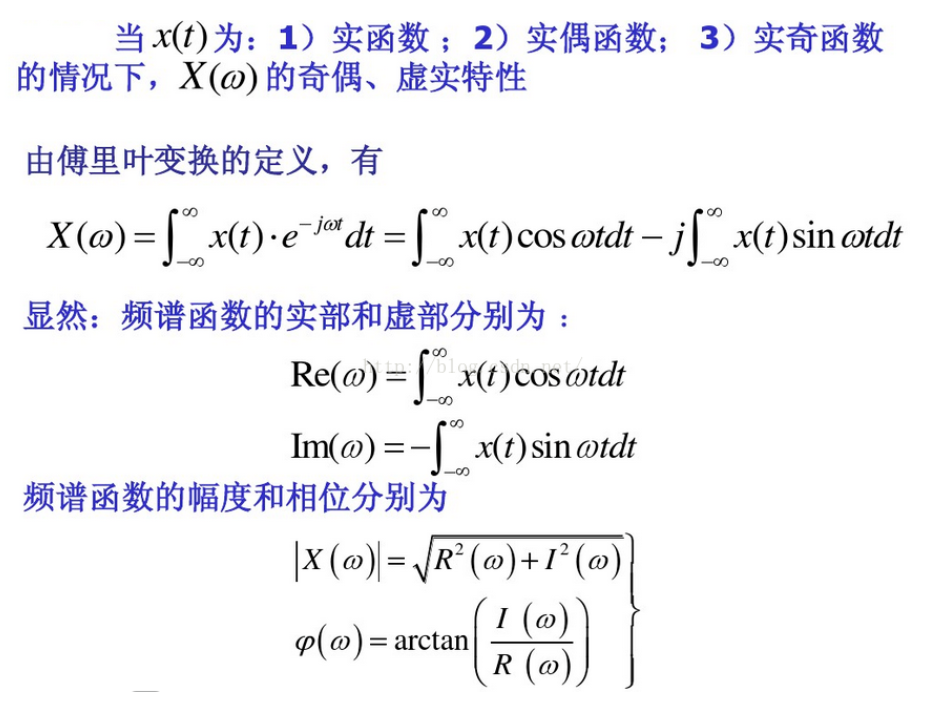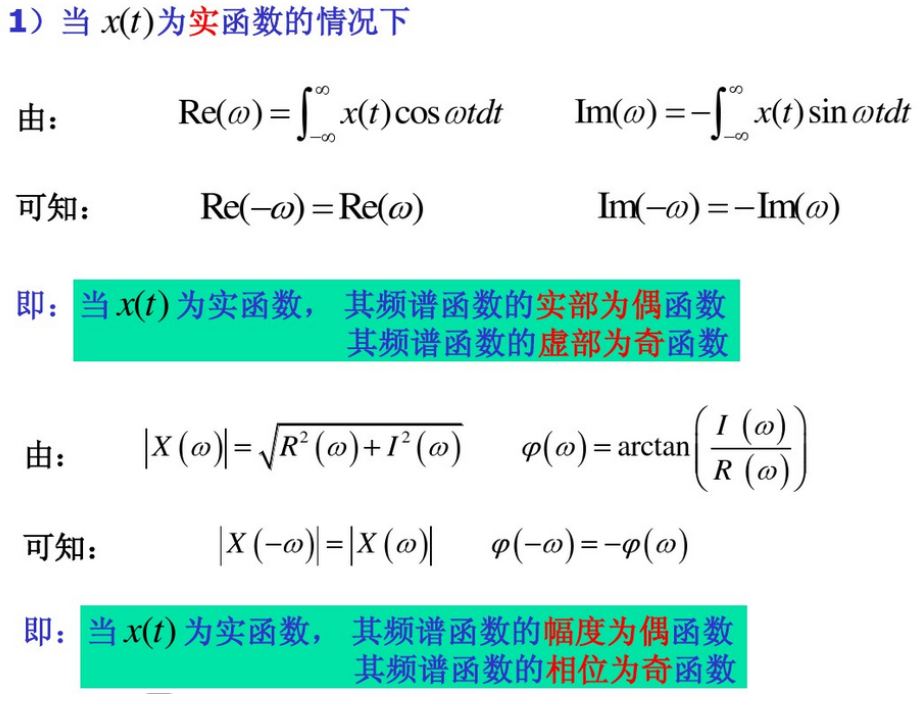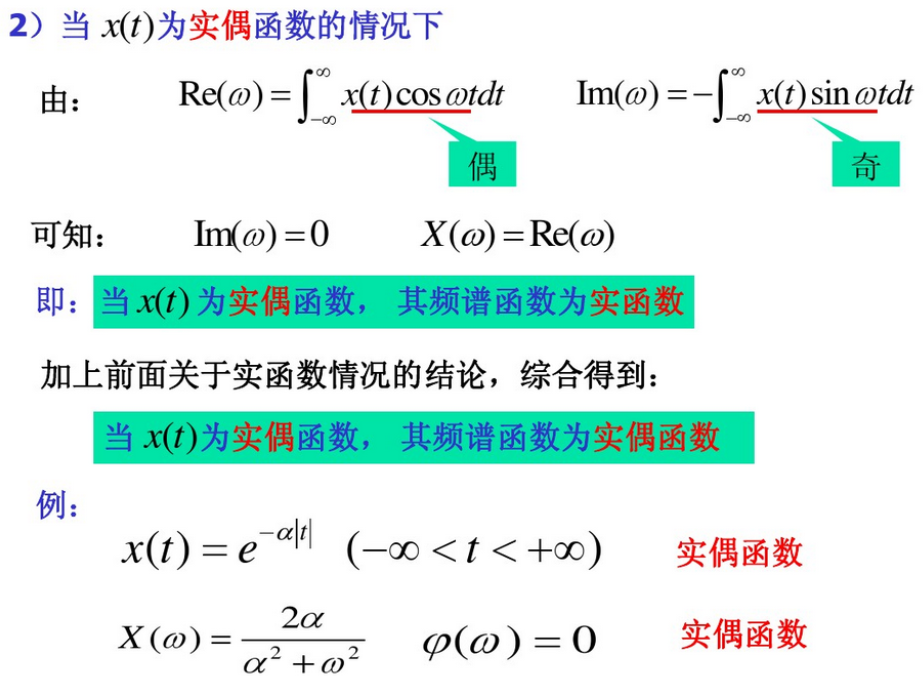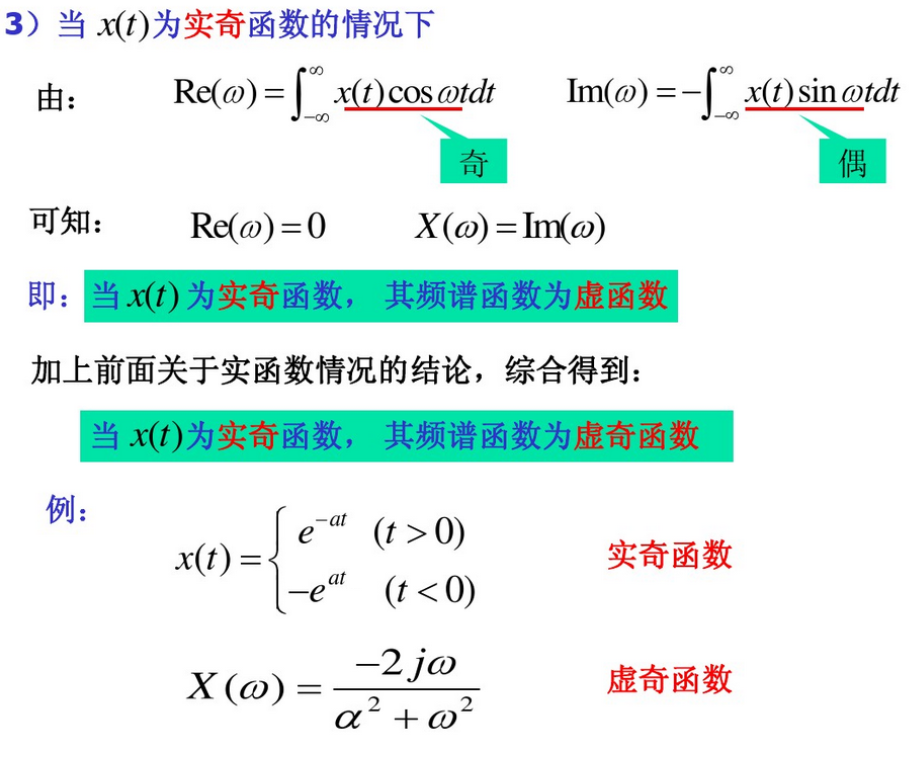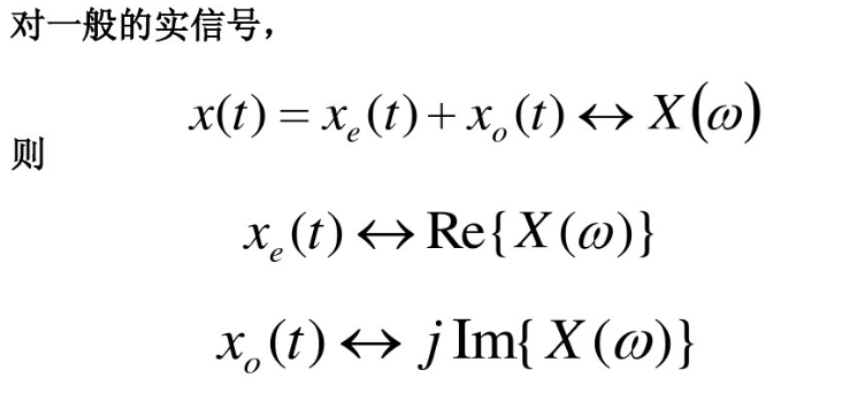详情请戳傅里叶变换–虚部的理解
傅里叶变换在opencv中实现图像去噪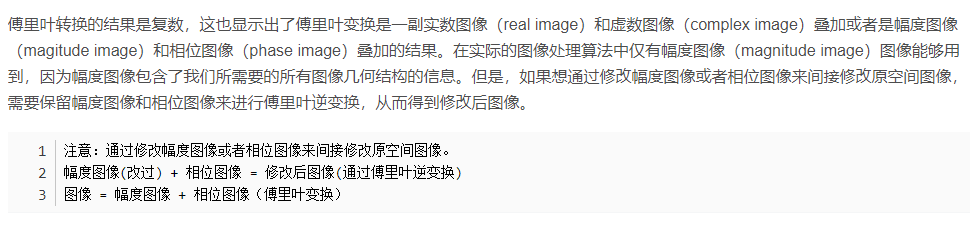代码演示：
#include "stdafx.h"
#include <opencv2\opencv.hpp>
#include <iostream>

#include<opencv2/opencv.hpp>
#include <iostream>
using namespace cv;
using namespace std;

int main()
{
if (src.empty())
{
return -1;
}

Mat srcGray;
cvtColor(src, srcGray, CV_RGB2GRAY); //灰度图像做傅里叶变换
imshow("Input Image", srcGray);

int m = getOptimalDFTSize(srcGray.rows); //2,3,5的倍数有更高效率的傅里叶变换
int n = getOptimalDFTSize(srcGray.cols);
cout << m << endl;
cout << n << endl;
//把灰度图像放在左上角,在右边和下边扩展图像,扩展部分填充为0;
copyMakeBorder(srcGray, padded, 0, m - srcGray.rows, 0, n - srcGray.cols, BORDER_CONSTANT, Scalar::all(0));
//这里是获取了两个Mat,一个用于存放dft变换的实部，一个用于存放虚部,初始的时候,实部就是图像本身,虚部全为零

Mat complexImg;
//将几个单通道的mat融合成一个多通道的mat,这里融合的complexImg既有实部又有虚部
merge(planes, 2, complexImg);

//对上边合成的mat进行傅里叶变换,***支持原地操作***,傅里叶变换结果为复数.通道1存的是实部,通道二存的是虚部
dft(complexImg, complexImg);
//把变换后的结果分割到两个mat,一个实部,一个虚部,方便后续操作
split(complexImg, planes);
//这一部分是为了计算dft变换后的幅值，傅立叶变换的幅度值范围大到不适合在屏幕上显示。高值在屏幕上显示为白点，而低值为黑点，高低值的变化无法有效分辨。为了在屏幕上凸显出高低变化的连续性，我们可以用对数尺度来替换线性尺度,以便于显示幅值,计算公式如下:
//=> log(1 + sqrt(Re(DFT(I))^2 +Im(DFT(I))^2))
//计算实数和虚数的幅值，并把幅值保存到palnes,允许在线覆盖
magnitude(planes, planes, planes_true);
Mat A = planes;
Mat B = planes;
Mat mag = planes_true;
//对幅值加1
mag += Scalar::all(1);
log(mag, mag);//计算出的幅值一般很大，达到10^4,通常没有办法在图像中显示出来，需要对其进行log求解。
//在这里进行一次低通滤波
for (int i = 0; i < mag.rows; i++)
{
for (int j = 0; j < mag.cols; j++)
{
float num = mag.at<float>(i, j);
if (num > 11.5)//13.5为幅度阈值，这里是低通滤波，高通滤波只需要改成＜就好
{
planes.at<float>(i, j) = 0;
planes.at<float>(i, j) = 0;
}
}
}
merge(planes, 2, complexImg);
//crop the spectrum, if it has an odd number of rows or columns
//修剪频谱,如果图像的行或者列是奇数的话,那其频谱是不对称的,因此要修剪
//这里为什么要用  &-2这个操作，我会在代码后面的 注2 说明
//我们知道x&-2代表x与 - 2按位相与，而 - 2的二进制形式是2的二进制取反加一的结果（这是补码的问题）。2 的二进制结果是（假设
//8位表示，实际整型是32位，但是描述方式是一样的，为便于描述，用8位表示）0000 0010，则 - 2的二进制形式为：1111 1110，
//x与 - 2按位相与后，不管x是奇数还是偶数，最后x都会变成一个偶数。
//就是说dft这个函数虽然对于输入mat的尺寸不做要求，但是如果其行数和列数可以分解为2、3、5的乘积，那么对于dft运算的速度会加快很多。
mag = mag(Rect(0, 0, mag.cols & -2, mag.rows & -2));
Mat _magI = mag.clone();
//这一步的目的仍然是为了显示,但是幅度值仍然超过可显示范围[0,1],我们使用 normalize() 函数将幅度归一化到可显示范围。
normalize(_magI, _magI, 0, 1, CV_MINMAX);
namedWindow("before rearrange", 0);
imshow("before rearrange", _magI);

//rearrange the quadrants of Fourier image
//so that the origin is at the image center
//重新分配象限，使（0,0）移动到图像中心，
//在《数字图像处理》中，傅里叶变换之前要对源图像乘以（-1）^(x+y)进行中心化。
//这是是对傅里叶变换结果进行中心化
int cx = mag.cols / 2;
int cy = mag.rows / 2;

//这里是以中心为标准，把mag图像分成四部分
Mat tmp;
Mat q0(mag, Rect(0, 0, cx, cy));   //Top-Left - Create a ROI per quadrant
Mat q1(mag, Rect(cx, 0, cx, cy));  //Top-Right
Mat q2(mag, Rect(0, cy, cx, cy));  //Bottom-Left
Mat q3(mag, Rect(cx, cy, cx, cy)); //Bottom-Right

q0.copyTo(tmp);
q3.copyTo(q0);
tmp.copyTo(q3);

q1.copyTo(tmp);
q2.copyTo(q1);
tmp.copyTo(q2);

normalize(mag, mag, 0, 1, CV_MINMAX);
imshow("spectrum magnitude", mag);

//傅里叶的逆变换
Mat ifft;
//傅里叶逆变换
idft(complexImg, ifft, DFT_REAL_OUTPUT);
normalize(ifft, ifft, 0, 1, CV_MINMAX);
imshow("inverse fft", ifft);
waitKey();
return 0;
}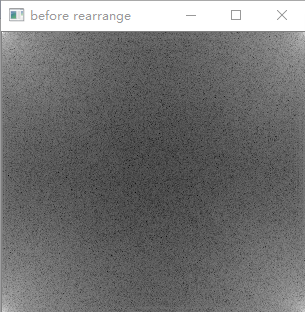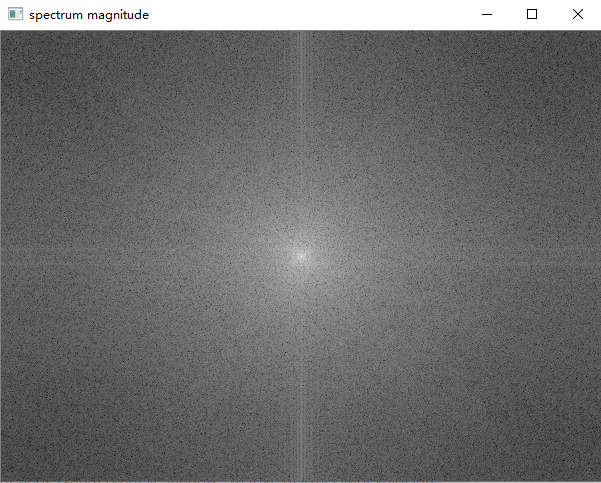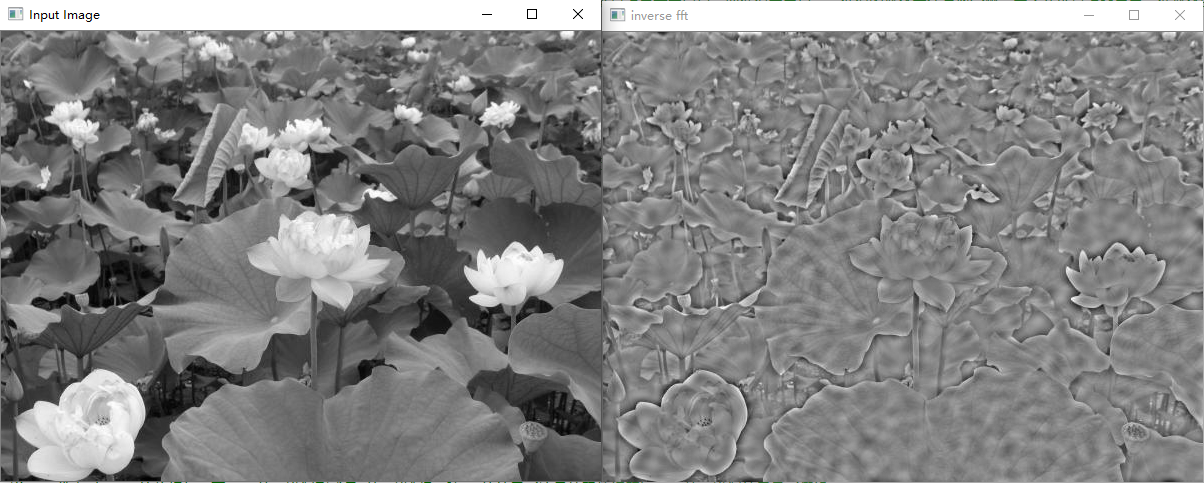展开全文• 一、一些关键概念的引入1、离散傅里叶变换（DFT）离散傅里叶变换(discrete Fourier transform) 傅里叶分析方法是信号分析的最基本方法，傅里叶变换傅里叶分析的核心，通过它把信号从时间域变换频率域，进而研究...
• 对LFM信号分数阶傅里叶变换后为什么会有两个峰值看看日历，情人节到，鲜花糖果，怎能少掉，满满爱意，将你拥抱，最美是你淡淡微笑。非平稳信号广泛存在于自然界与现实生活中，作为非平稳信号的线性调频信号(Liner ...
• ## 傅里叶变换(二维离散傅里叶变换)

万次阅读 多人点赞 2018-06-15 22:22:35
离散二维傅里叶变换一常用性质： 可分离性、周期性和共轭对称性、平移性、旋转...根据快速傅里叶变换计算要求，需要图像的行数、列数均满足2的n次方，如果不满足，在计算FFT之前先要对图像补零以满足2的n次。 ...
• Q:简述计算机三大变换的联系和区别 (傅里叶变换 拉普拉斯变换 z变换)（1） 傅里叶变换定义：表示能将满足一定条件的某个函数表示成三角函数（正弦和/或余弦函数）或者它们的积分的线性组合。傅立叶变换是一种...计算机数学
• ## 从傅里叶变换到加窗傅里叶变换

万次阅读 多人点赞 2017-12-10 14:00:49信号与系统 信号处理
• 滑动离散傅立叶变换（SDFT）在计算上非常有效，并且在其标称频率下工作时能够提供出色的谐波抑制性能。 但是，在标称频率之外，幅度和相位角都包含由于频谱泄漏引起的误差。 而且，在这种情况下，它的谐波抑制能力...matlabFFT
• 文章目录傅里叶变换基础傅里叶级数傅里叶积分傅里叶变换一维连续傅里叶变换一维离散傅里叶变换二维离散傅里叶变换变换变换卷积卷积定理数字图像DFT空间域和频域图像频域滤波基本步骤图像频率特性分析图像滤波...
• ## OpenCV中的图像变换——傅里叶变换

万次阅读 多人点赞 2021-07-22 20:11:24
这篇博客将介绍OpenCV中的图像变换，包括用Numpy、OpenCV计算图像的傅里叶变换，以及傅里叶变换的一些应用；python opencv
• 一维傅里叶变换数学推导 傅里叶级数，形如： 是由三角函数系 1，cosx，sinx，cos2x，sin2x，…，cosnx，sinnx，…(2) 经过组合所产生的三角级数。a0，an，bn 称为傅里叶系数。为了求傅里叶系数，我们先引出...计算机视觉 python
• 利用傅里叶变换求得音频数据的频率，根据音高频率对照表，可测试出吉他每根弦与标准音的差值，实现调音器效果。 目录 程序效果 实现过程 样例代码 测试用例 参考资料 程序效果 截图1：程序效果 输入...调音器 C语言 FFT
• 注：本文为博主参考书籍和他人文章并加上自己的理解所编，作为学习笔记使用并将其分享出去供大家学习。若涉及到引用您的文章内容请评论区告知！...一、什么是傅里叶变换   时域及频域  在讲...数学 图像处理 欧拉公式
• 傅里叶变换可以看作是数学上的棱镜，将函数基于频率分解为不同的成分。当我们考虑光时,讨论它的光谱或频率谱。同样, 傅立叶变换使我们能通过频率成分来分析一个函数。 Fourier theory讲的就是：任何信号...
• 连续傅里叶变换 傅里叶变换的性质 离散傅里叶变换(DFT) 从前面我们已经知道，非周期连续函数傅里叶变换如下 F(ω)=∫−∞+∞f(t)e−iωtdt F(\omega)=\int ^{+\infty}_{-\infty}f(t)e^{-i\omega t}dt F(ω)=∫−...算法 卷积
• 在上一篇随笔“使用快速傅里叶变换计算大整数乘法”中，已经讲述了使用快速傅里叶变换计算大整数乘法的原理。在这一篇随笔中，我们就使用快速傅里叶变换来实现一个提供任意精度的算术运算的静态类：BigArithmetic。 ...
• 傅里叶变换的意义在于提取一张图片的频率成分，理论推导见笔记本。 映射关系 其映射关系可以大致总结成 f(x,y)−−−>F(u,v) 其中图片可以看作是一个矩阵，矩阵里的元素是灰度值。而矩阵又可以看作是函数的...
• ## 傅里叶变换

千次阅读 2019-07-20 16:17:57
此篇博文主要简述傅里叶变换的相关概念以及如何代码实现离散序列的傅里叶变换 我们都知道，傅里叶变换是频域分析的重要工具；其将信号从时域转换到了频域，以更直观的角度向我们展示了信号的本质——频率。 下面不加...自动控制原理 信号处理与分析 频域分析
• 在信号处理领域，存在诸多变换，比如标题中的五个变换。本文将对这五个变换进行介绍和比较。在开始之前，我们需要先理清什么是...通常傅里叶变换只适合处理平稳信号，对于非平稳信号，由于频率特性会随时间变化，为了信号处理 数字信号处理 matlab 小波分析
• C#代码，FFT计算过程，傅里叶变换，虚拟示波器产品项目实测结果可靠。FFT
• 3. 图像经过傅里叶变换频率中心化后能够容易地复原； 从而进一步说明图像通常需要进行频率中心化操作以方便处理； 1.大部分图片的有效信息集中在低频部分 图片的高频部分主要是边缘等细节，主要内容大部分是...数字图像处理
• 而正是所谓的“傅里叶变换”使得我们可以工作于频率域。 一维连续函数的fourier变换 其中，f(x)表示原函数，F（u）表示变换之后的函数。u为频率域变量。 一维连续函数的fourier反变换 。。。...
• 一 四个名词：实际物理频率，角频率，圆周频率，归一化频率，数字频率，模拟频率 ...设置角频率纯粹为了便于计算。） 3、归一化频率是将实际物理频率按fs归一化之后的结果，最高的信号频率为fsfft...